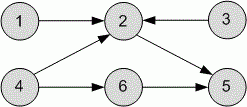Competitions

Topological Sort

The directed unweighted graph is given. Sort topologically its vertices.

Input

The first line contains the number of vertices n (1n105) and the number of edges m (1m105) in a graph. Each of the next m lines describes the edge of the graph - two numbers, the initial and final vertex.

Output

Sort the graph topologically and print its sequence of vertices. If its impossible to sort graph topologically, print -1.Time limit 2 seconds
Memory limit 128 MiB
Input example #1
6 6
1 2
3 2
4 2
2 5
6 5
4 6
Output example #1
4 6 3 1 2 5
Author Vitaly Goldstein
Source Winter School, Kharkov, 2011, Day 9